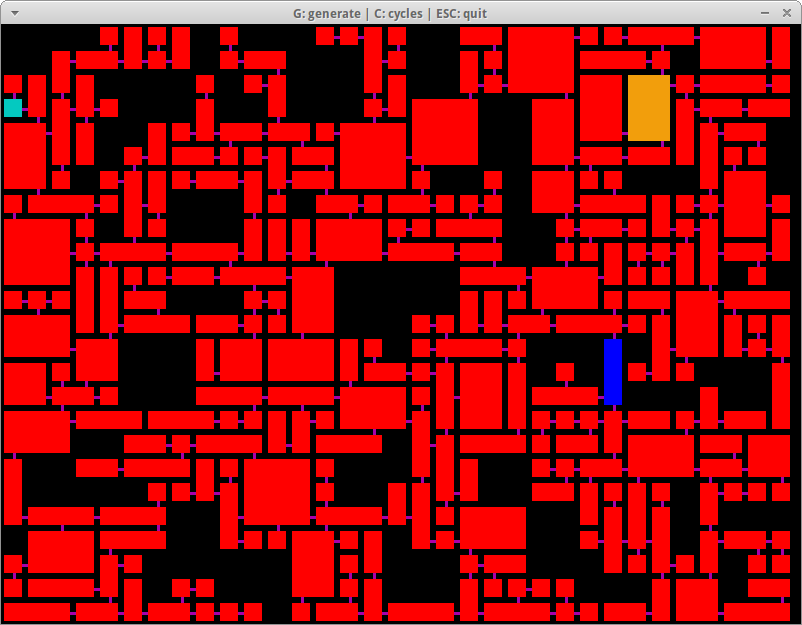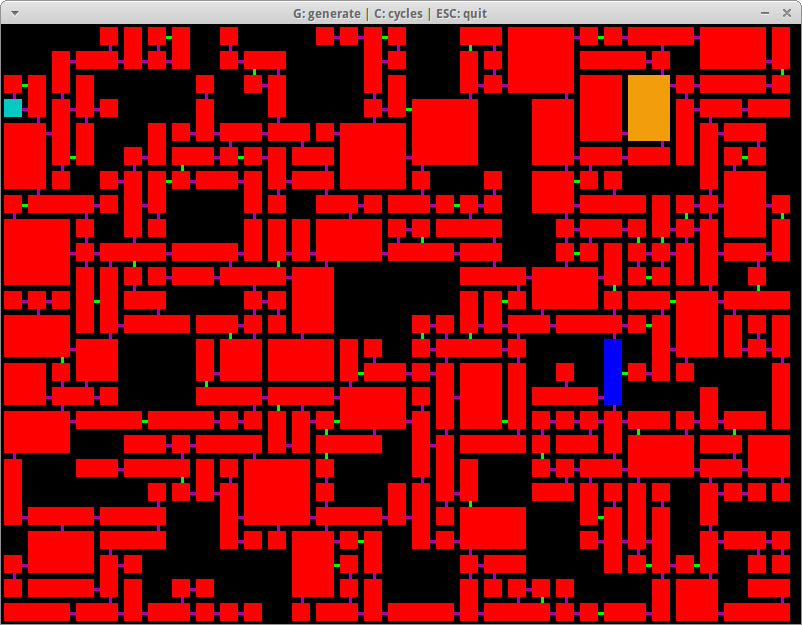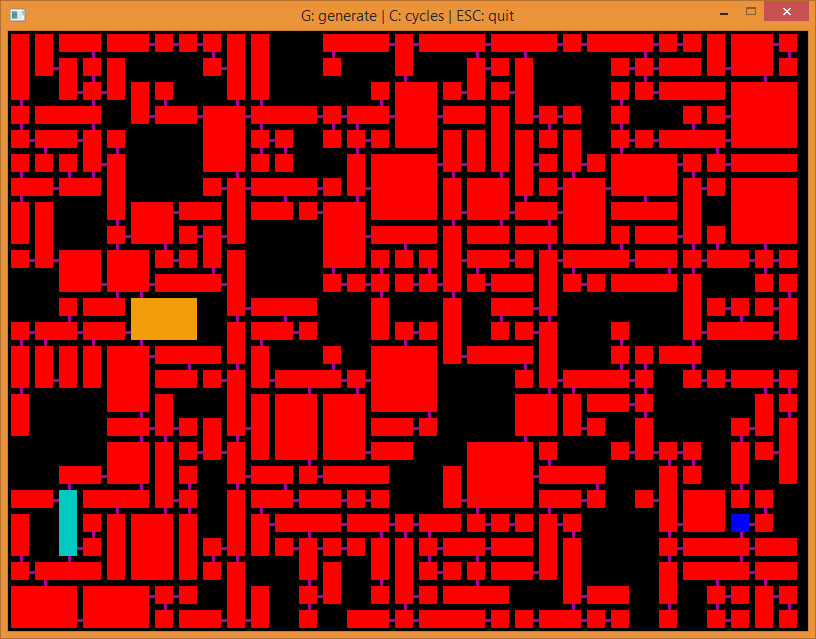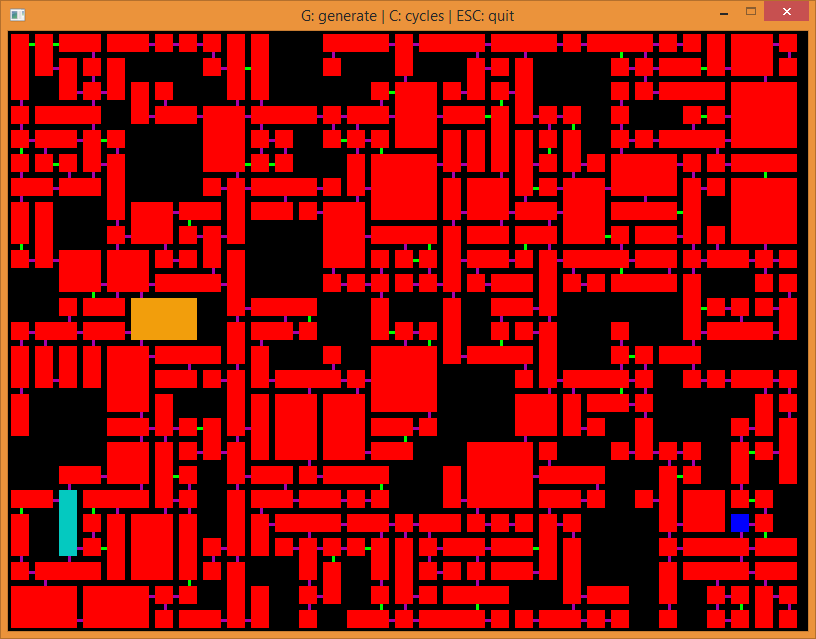# Generating a Dungeon (part 3)

When I last left off I had the rooms (or data equivalent of rooms) created, the last thing that needed to be done was add in cycles so that the dungeon layout is not a tree. That’s what I’ll be tackling in this post.

I’ve updated the `generateMap()` function and added a call to the new function `fixTree()` at the end:

`fixTree()`

```void GeneratedMap::fixTree()
{
for (unsigned int i = 0; i < rooms.size(); i++)
{
}

unsigned int i = 0;
//keep adding cycles until the quantity is met or we run out of
{
{
continue;
}

unsigned int j = 0;
Room* aj = nullptr;
//find first non-connected room
do
{
j++;
} while (j < adjacentRooms.size() && aj == nullptr);

//if no non-connected room is found try the next dead-end
if (aj == nullptr)
{
continue;
}

aj->hasSecondEntrance = true;

//rooms are adjacent, but not connected, now we connect them... with
//wizardry...
int directionOfOther = de->directionOfOtherRoom(aj);
int miny = 0, minx = 0, shift = 0;
if (directionOfOther == Room::LEFT || directionOfOther == Room::RIGHT)
{
miny = std::max(de->topLeft.y, aj->topLeft.y);
int maxy = std::min(de->bottomRight.y, aj->bottomRight.y);
int dy = std::abs(maxy - miny);
shift = dy <= 0 ? 0 : rand() % (dy + 1);
}
else if (directionOfOther == Room::UP || directionOfOther == Room::DOWN)
{
minx = std::max(de->topLeft.x, aj->topLeft.x);
int maxx = std::min(de->bottomRight.x, aj->bottomRight.x);
int dx = std::abs(maxx - minx);
shift = dx <= 0 ? 0 : rand() % (dx + 1);
}

switch (directionOfOther)
{
case Room::LEFT:
aj->startingCell2 = Vector2i(aj->bottomRight.x, miny + shift);
aj->previousCell2 = Vector2i(de->topLeft.x, miny + shift);
break;
case Room::RIGHT:
aj->startingCell2 = Vector2i(aj->topLeft.x, miny + shift);
aj->previousCell2 = Vector2i(de->bottomRight.x, miny + shift);
break;
case Room::UP:
aj->startingCell2 = Vector2i(minx + shift, aj->bottomRight.y);
aj->previousCell2 = Vector2i(minx + shift, de->topLeft.y);
break;
case Room::DOWN:
aj->startingCell2 = Vector2i(minx + shift, aj->topLeft.y);
aj->previousCell2 = Vector2i(minx + shift, de->bottomRight.y);
break;
}

}
}
```

The method that’s called before that, `fillRooms()` adds in all the rooms and their connections. It uses a “flood fill” type algorithm to fill the dungeon with rooms. An individual room has four named pointers to child rooms that spawn from it: `left, right, bottom,` and `top`. Consequently we can detect when a room is a dead end by checking if all of its child pointers are null:

```bool Room::isDeadEnd()
{
return !left && !right && !bottom && !top;
}
```

This is what the `fixTree()` function starts with, a list of dead ends:

```//get all dead ends
for (unsigned int i = 0; i < rooms.size(); i++)
{
}
```

The plan here is to take this list of dead-ends and for a certain percent of them (in my code I used 50%), try to find an adjacent room that isn’t connected to it, then connect the two. In the code I added `startingCell2` and `previousCell2` to the `Room` class. These values are assigned on the adjacent room.

After finding the dead-ends, we set up the conditions for iterating through them:

```unsigned int j = 0;
//keep adding cycles until the quantity is met or we run out of
{
```

For each dead-end we’re iterating through, start by getting a list of all adjacent rooms (non-diagonal):

```Room* de = deadEnds[j++];
{
continue;
}
```

The `getAdjacentRooms()` function creates a list of `Room` pointers, then looks at all the cells along the left, right, top, and bottom side of the dead-end room in order, and adds all the rooms occupying those cells into that list. I thought this function would be a good place to try out lambdas that were added in C++11. I’m familiar with C# lambdas and callbacks, but haven’t used them in C++ until now. The code I put inside of it needed to be used in four different places in the `getAdjacentRooms()` function, but it didn’t feel like it warranted its own function.

`getAdjacentRooms()`

```std::vector<Room*> GeneratedMap::getAdjacentRooms(Room* room)
{

//a lambda that will make the code below shorter
//it checks if the given room is not null, if it isn't, adds the given
//room to the roomsToCheck vector.
-> void
{
if (room == nullptr)
return;

bool found = false;
for (int i = 0; i < adjacentRooms.size(); i++)
{
{
found = true;
break;
}
}

if (!found)
};

//rooms to left
if (room->topLeft.x > 0)
{
int x = room->topLeft.x - 1;
for (int y = room->topLeft.y; y <= room->bottomRight.y; y++)
}

//rooms to right
if (room->bottomRight.x < myWidth - 1)
{
int x = room->bottomRight.x + 1;
for (int y = room->topLeft.y; y <= room->bottomRight.y; y++)
}

//rooms to top
if (room->topLeft.y > 0)
{
int y = room->topLeft.y - 1;
for (int x = room->topLeft.x; x <= room->bottomRight.x; x++)
}

//rooms to bottom
if (room->bottomRight.y < myHeight - 1)
{
int y = room->bottomRight.y + 1;
for (int x = room->topLeft.x; x <= room->bottomRight.x; x++)
}

}
```

Back in the while loop of the `fixTree()` function, after the list of adjacent rooms is created, we try to find the first room in the list that’s not already connected to the dead-end. If we do find one, mark that room as having a second entrance, otherwise move on to the next dead-end:

```unsigned int j = 0;
Room* aj = nullptr;
//find first non-connected room
do
{
j++;
} while (j < adjacentRooms.size() && aj == nullptr);

//if no non-connected room is found try the next dead-end
if (aj == nullptr)
{
continue;
}

aj->hasSecondEntrance = true;
```

The `areConnected()` function checks the Room pointers on both rooms and makes sure that none of them are the other room in question. That explanation is terrible, so here’s the code:

```bool Room::areConnected(Room* other)
{
//this function got long during debugging, decided to keep it that way

bool lefta = left != nullptr && left == other;
bool righta = right != nullptr && right == other;
bool topa = top != nullptr && top == other;
bool bottoma = bottom != nullptr && bottom == other;
if (lefta || righta || topa || bottoma)
return true;

bool leftb = other->left != nullptr && other->left == this;
bool rightb = other->right != nullptr && other->right == this;
bool topb = other->top != nullptr && other->top == this;
bool bottomb = other->bottom != nullptr && other->bottom == this;
if (leftb || rightb || topb || bottomb)
return true;

return false;
}
```

If a non-connected adjacent room has been found and marked as having a second entrance, we then figure out what the x and y values are for `previousCell2` and `startingCell2` for that room. First we figure out the direction of that room.

```int Room::directionOfOtherRoom(Room* other)
{
if (other->bottomRight.x < topLeft.x)
return LEFT;

if (other->bottomRight.y < topLeft.y)
return UP;

if (other->topLeft.x > bottomRight.x)
return RIGHT;

if (other->topLeft.y > bottomRight.y)
return DOWN;

return 0;
}
```

We then need to decide where this entrance is going to be placed, this is done with the following code:

```int directionOfOther = de->directionOfOtherRoom(aj);
int miny = 0, minx = 0, shift = 0;
if (directionOfOther == Room::LEFT || directionOfOther == Room::RIGHT)
{
miny = std::max(de->topLeft.y, aj->topLeft.y);
int maxy = std::min(de->bottomRight.y, aj->bottomRight.y);
int dy = std::abs(maxy - miny);
shift = rand() % (dy + 1);
}
else if (directionOfOther == Room::UP || directionOfOther == Room::DOWN)
{
minx = std::max(de->topLeft.x, aj->topLeft.x);
int maxx = std::min(de->bottomRight.x, aj->bottomRight.x);
int dx = std::abs(maxx - minx);
shift = rand() % (dx + 1);
}
```

Now the above code looks kind of cryptic, so I’ll go into more detail about what it does.

It finds the range of cells that the entrance can be placed in and picks a value. For example, if rooms are next to each other on the left and right, there are three scenarios: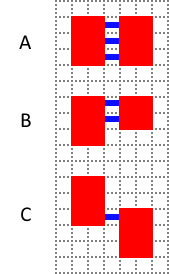A) The two room’s top and bottom cells line up exactly.
B) The top/bottom cells of one room are inside the top/bottom cells of another
C) The two rooms partially overlap, so that each has Y axis cells that don’t line up with the other.

What we need to do is find a “safe range” of Y axis values that are in both rooms. This is what `miny` and `maxy` are used for: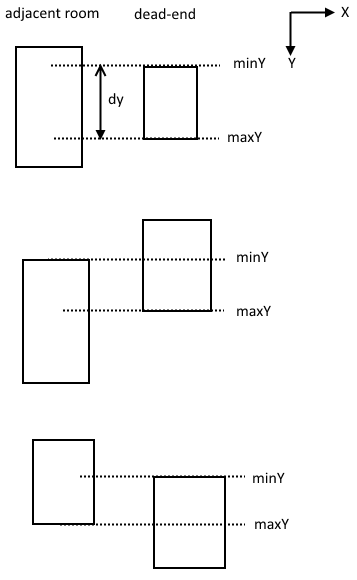This picture illustrates what the code is doing. It takes the higher Y value of both top-cells and the lower value of the bottom cells of the rooms gives us a vertical range that is guaranteed to contain both rooms.

Also notice that in scenario A and B there are multiple choices for which Y axis value to choose when connecting the dead-end to the adjacent room. That’s where the `dy` and `shift` values come into play. The difference in height, `dy`, is found by taking the absolute value of the difference of the min and max Y values, in math terms:$\Delta y = | y_{max} - y_{min} |$.

If the `dy` is 0, (ie: both rooms are one cell tall and match on their top/bottom cells), then the shift needs to be 0. If `dy` is greater than 0, then a value between 0 and `dy`, inclusive, must be chosen. Modulus operations in the form of `x mod y` have a range between 0 and y-1 inclusive, in math terms:$[0,y)$. So we can still get what we want in both cases by adding 1 to `dy`.

All of this is happens on the X axis as well if needed, hence the two if statements.

After the entrance is chosen the cell locations just need to be stored in the adjacent room, the number of connections added needs to be incremented, then go back to the top of the while loop:

```switch (directionOfOther)
{
case Room::LEFT:
aj->startingCell2 = Vector2i(aj->bottomRight.x, miny + shift);
aj->previousCell2 = Vector2i(de->topLeft.x, miny + shift);
break;
case Room::RIGHT:
aj->startingCell2 = Vector2i(aj->topLeft.x, miny + shift);
aj->previousCell2 = Vector2i(de->bottomRight.x, miny + shift);
break;
case Room::UP:
aj->startingCell2 = Vector2i(minx + shift, aj->bottomRight.y);
aj->previousCell2 = Vector2i(minx + shift, de->topLeft.y);
break;
case Room::DOWN:
aj->startingCell2 = Vector2i(minx + shift, aj->topLeft.y);
aj->previousCell2 = Vector2i(minx + shift, de->bottomRight.y);
break;
}